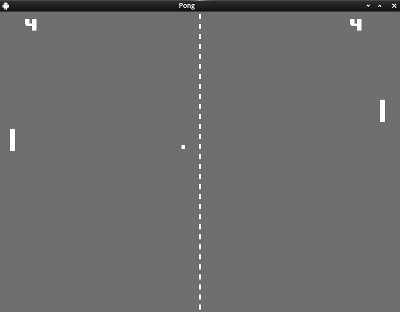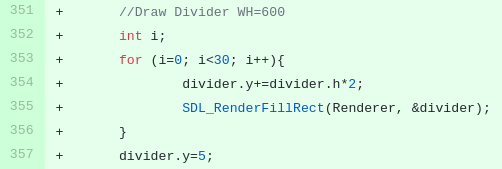# How to create multiple SDL_Rects from one

I want to make a breakout clone in SDL2. I want to make one SDL_Rect and cloning it in different coordinates. Any help would be appreciatedAnother solution would be the declaration of multiple rects in the globals.

`SDL_Rect divider;`

Initialize them in your set/init game:

``````	for (int i=0; i<30; i++){
divider[i].w =   4;
divider[i].h =  10;
divider[i].x = 398;
divider[i].y = 5+i*(divider[i].h*2);
}
``````

and finally render them:

``````for (int i=0; i<30; i++){
SDL_RenderFillRect(Renderer, &divider[i]);
}
``````

-Cass

But seriously, create an github account, fork my project and work there.